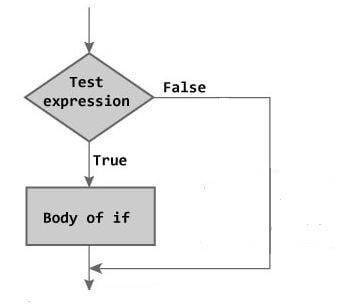# if statement in C

The if statement is one of the selection statements.  It uses to select statements depending on the value of a controlling expression.

Syntax:

if ( controlling expression )
statement;

In the above scenario, the statement will only be executed if the controlling expression is non-zero.

Note: statement could be a single statement or compound statement.  A Compound or block statement is a group of the statements within curly braces “{}”.  All statements of the compound statement are executed sequentially.Let see  an example,

The below program check even number. If a number is divided by 2, it means it is an even number.

#### How the above program works:

1 Case: When you entered even number:

We know that the modular division of an even number by 2 is 0. So expression (data % 2) return 0, as we know expression ( 0 == 0) return 1.

Now  if((data % 2) == 0) become if(1), as per C standard if the controlling  expression is  non-zero then body associated with if will be execute. So statement printf(“\n Number is Even.\n\n”) will be execute.

2 Case: When you entered odd number:

For odd number expression (data % 2) return 1, so  expression ( 1== 0) return 0.
Now if((data % 2) == 0) become if(0), so body of if will be skip and no message will be display on console.

#### Some important points need to remember before using the if

1. Don’t put semicolon just after the if(expression). If you put the semicolon after the if selection statement, then it has no meaning to write if selection statement in a program.

See below code,

2. When only one statement belongs to an if statement, then braces are optional.

See the below codes,

3. A non-zero value is considered true and zero (0) is considered false in C.

4. In place of controlling expression, we can also call the function that returns a true or false value.

For example,

if(!strcmp(str1,str2))
{

//body

}

5. Using the logical operator we can use more than one condition with if selection statement.